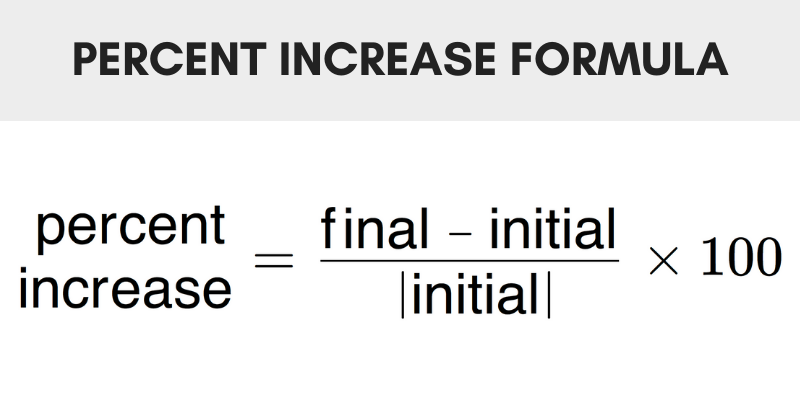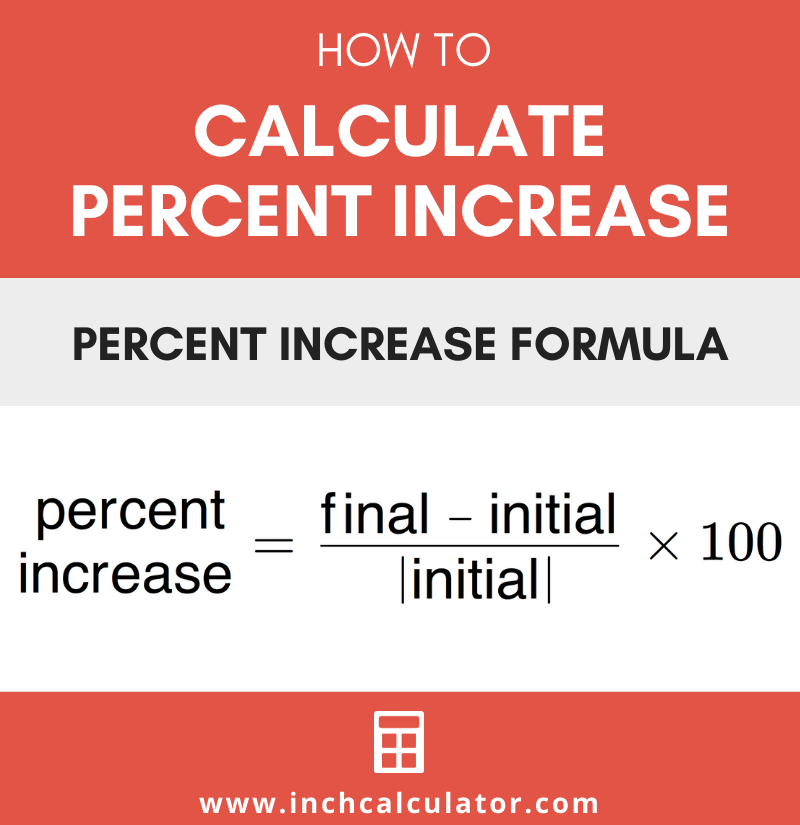# Percent Increase Calculator – Find Percentage Increase

Find the percent increase or the growth in a value by entering the known values below.

%

## Result:

40% increase

### Steps to Solve Percent Increase

p=(y2 - y1) / |y1|×100
Substitute values in the equation
p=
(105 - 75) / |75|×100
Simplify
p=
30 / 75×100
p=0.4×100
p=40%
Learn how we calculated this below

## How to Find Percent Increase

Percent increase is the growth in a value expressed as a percentage. It is the percent of change from the initial value to the final value.

To calculate the percentage increase of a value, use the following formula.

### Percent Increase Formula

The percentage of increase is defined as the difference between the initial value and final value divided by the initial value. The percent increase formula is:

p = final – initial / |initial| × 100

Thus, the increase in value expressed as a percentage is equal to the final value minus the initial value, divided by the absolute value of the initial, times 100.For example, let’s calculate the percentage increase from 50 to 80.

p = 80 – 50 / |50| × 100
p = 30 / 50 × 100
p = 0.6 × 100
p = 60% increase

## How to Calculate the Growth by a Percentage

If the percent increase is known, you can calculate the growth of a value to the final value by multiplying the initial value by the percentage and adding that to the initial value.

final = (initial × p / 100) + initial

Thus, the final value is equal to the initial value times the percentage divided by 100, plus the initial value.

For example, let’s calculate the growth of the initial value 80 by 50%

final = (80 × 50 / 100) + 80
final = (80 × .5) + 80
final = 40 + 80
final = 120

Use our percent decrease calculator to find the decrease in a value.